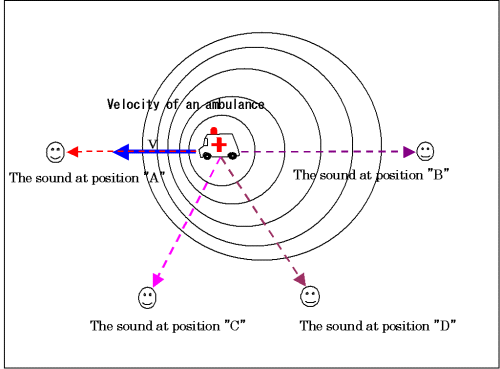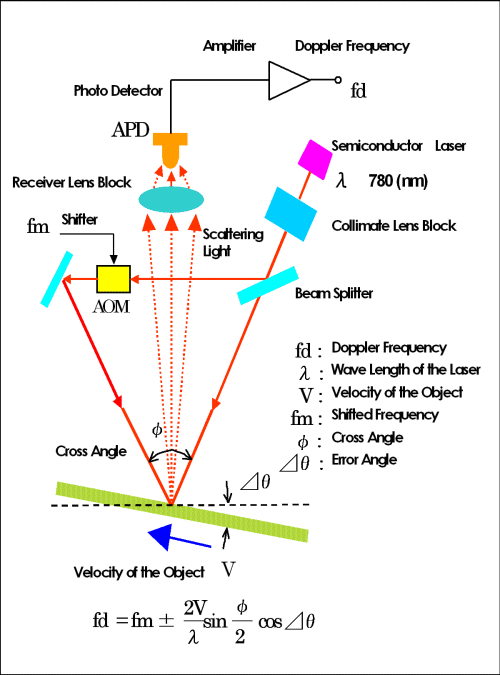# Technology

### 1. What is the Doppler effect ?Fig1. What is the Doppler effect ?

The Doppler effect (Doppler effect) means the phenomenon in which the wavelength (frequency) of the wave which a watcher measures differs compared with it in a generation source, when the velocity difference relative between the generation source of a wave (sound, light, and electric wave) and a watcher has arisen.

For example, as shown in the above figure, in the front of a generation source, the number of the waves which arrive within a fixed time increases the frequency of the generation source which is moving — on the other hand behind a generation source, the number of the waves which arrive within fixed time decreases.

It was named by the physicist “Doppler, Johan Christian (1803 to 1853)” of Austria who studied this effect, and this phenomenon was found by him first in 1842.

The above figure should explain a little in more detail. Consider signs that it has approached while an ambulance sounds a siren. When it is moving at velocity with a sound source and the watcher has stopped, a siren’s sound, which a watcher hears is influenced by the velocity of a sound source.

The watcher A hears a siren’s sound in the front of an ambulance and hears the sound of wavelength shorter than a sound source. B hears behind an ambulance and hears wavelength longer than a sound source. This is because the sound wave received “frequency shift” by Doppler effect.

The relation C and D is also same as the case of A and B.

The Doppler effect which we have experienced in everyday life is this one.

Our Laser Doppler sensor to be explained from now on has taken the method of detecting velocity from the direction of sideways, like the relation of C and D.

This is the sensor which had the feature of detecting the amount of shifts contained in scattering light by differential from a transverse direction.

That is greatly different from the vibro-meter which detects velocity from right reflecting light.

### 2. The composition and the measurement principle of the Laser Doppler sensor.Fig2. Principal and Construction of the Doppler Sensor

The basic composition of Laser Doppler sensor puts two irradiation light on a front [ of the advance direction of object ], and back side, and has the form where scattering light reflected from object, respectively is received in one optical receptor. In this scattering light, the velocity information on a measured object is contained in the form of wavelength change of light. Although the dispersion light from each irradiation light is changing in the direction where wavelength becomes short in a front side, and the direction which becomes long in a back side, it carried out heterodyne detection of the difference of the mutual wavelength, and has detected velocity. K01, K02 are vectors. fD1,fD2 are frequency differences between irradiation lights and reflection light.
fD1 = V * (K- K01)
fD2 = V * (K+ K02) can be shown as above.
When K01, K02 are received on an optical receptor, fD1- fD2 is a “beat frequency” from the measured object.
fD = | fD1-fD2| = V * (K01+ K02)
Above shows vector K will loose which shows a direction of receptor and fD is created by the direction of the irradiation lights and the moving object. This composition is called “Differential composition” .

“K01= K02” makes as K01= ( ) * cos fD = 2V * K01= ( ) * cos

fD is showed by angle of intersection. cos = cos ( – ) = sin ()

fD = * sin () and add the error angle of the object as.

fD = * sin () * cos

As above equation shows that the velocity of object V can be created by Doppler frequency fD.

### 3. Features

The non-contact Laser Doppler Velocity Meter using the laser which has many features as follows

1. There is no physical influence on a measured object.Non-contact measurement maintains the object completely unaffected and allows the user to measure an object without disturbing the physical phenomenon and without applying any load. This is the advantage of the optical technique. Velocity of the thin film and paper which were not able to be measured by the technique of a contact type like a rotary encoder. Velocity of the metal plate of hot-rolling which is high temperature. Measurement of the velocity of the small mechanism which cannot attach a sensor because of measurement etc. was attained.
2. A wide range of objects can be measured.If iron, nonferrous metal, various kinds of resin, a tree, paper, cloth, glass, ceramics, etc. Reflect dispersion light, they can be measured anything. Moreover, that by which the surface was printed, and the thing applied in various colors can also be measured.
3. Easy to set and operate.
In order to take out velocity information out of scattering light, strict alignment adjustment is not required. It can measure, if only a measured object is in the measurable depth of a sensor.